# Event Structures as Presheaves

#### Supervised by:   Ohad Kammar

University of Oxford, 18 June - 24 August 2018

# I.A. Historical Example

« […] you can build a mind from many little parts, each mindless by itself. »
Marvin Minsky, The Society of Mind (1986)

Mental agents:
small processes $\begin{cases} \text{ making up the human mind } \\ \text{ each of which performs a very simple and specific task } \end{cases}$
Society of Mind:
All the mental agents interactions ⟹ Intelligence
Marvin Minsky (1927-2016)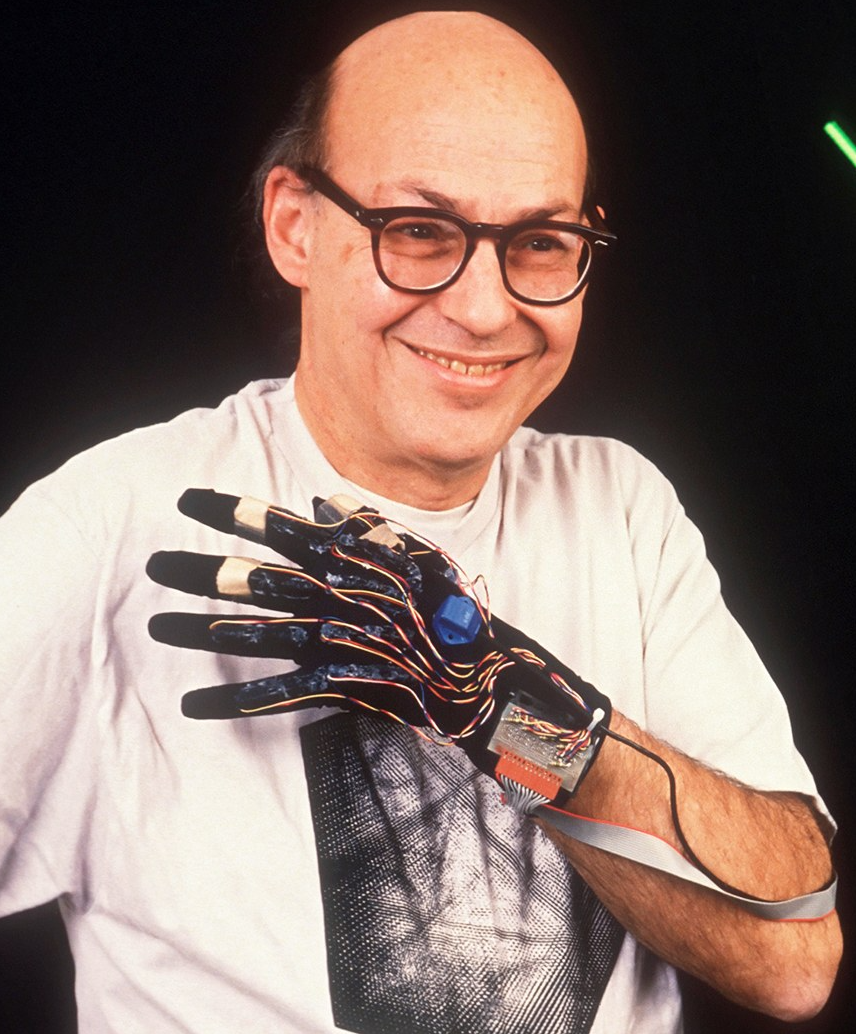A.I. Researcher & Cognitive Scientist (MIT):
• A.I. Pioneer with John McCarthy
• Left his mark on popular culture:
• Adviser on Kubrick's 2001: A Space Odyssey
• Even appears in Digimon!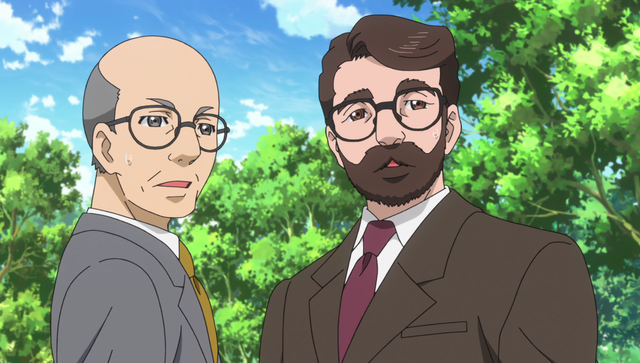# Minsky’s toy example## Playing with Blocks (Pb)

Calls two simpler mental agents:

### And when he's done Playing with his Blocks (Pb)   and   with his Dolls (Pd)## ... he'd better tidy up! (T)

The mental agents here form an example of event structure:… which can also be seen as a non-deterministic process:

choose 'Pb' or 'Pd' or ('Pb' and 'Pd'):
if 'Pb':
choose either 'B' or 'W':
if 'Pb' and 'Pd':
launch 'T'


Event Structures:

• Domain theory Programming Language Semantics

• Category Theory Algebraic Effects

# II. Event Structures as Domains

Well-founded poset   $E \eqdef \pair{\carrier E}{\leq_E}$:
poset such that $∀ \, e\in \carrier E, \quad \dc e \eqdef \underbrace{\lbrace e'\in\carrier E \mid e' \leq_E e \rbrace}_{\text{downward closure of } e}$     is finite.

Event structure   $E \eqdef \triple{\carrier E}{\leq_E}{\Con[E]}$:
1. a well-founded poset $(\carrier E, \underbrace{\leq_E}_{\rlap{\text{causal dependency relation}}})$ of events

2. a consistency relation $\Con[E] \subseteq \Pfin(E)$ such that:

• $∀ \, e\in \carrier E, \quad \lbrace e \rbrace \in \Con[E]$
• closure under subsets:     $X \subset Y \in \Con[E] \implies X \in \Con[E]$
• augmentation property:     $x \in \Con[E] \; ∧ \; e' \leq_E e \in x \; ⟹ \; x \cup \lbrace e' \rbrace \in \Con[E]$

### Playing child example

All the subsets of $\carrier E ≝ \lbrace {\rm Pb}, {\rm Pd}, \rm B, \rm T, \rm W\rbrace$ are in $\Con[E]$ except those containing $\lbrace \rm W, \rm B \rbrace$, or $\lbrace \rm W, \rm T \rbrace$.Crucial concept:

Configurations = event history of a partial run of our computational process.

For that, we need two additional notions:

### 1. Consistent subsets

$$\carrier E \supseteq x \text{ is consistent} \iff \begin{cases} x∈\Con[E] &\text{x is finite} \\ \Pfin(x) \subseteq \Con[E] &\text{otherwise} \end{cases}$$Extends the definition of ‘being in the consistency relation’ to infinite subsets

### 2. Down-closed subsets:

$$x \subset \carrier E \text{ is down-closed } \; ⟺ \; \dc x \eqdef \bigcup_{e\in x} \dc e \subseteq x$$$x$ contains all the events that must have happened for any $e ∈ x$ to occur.

### Configurations

Set of configurations:
$$\ConfStar[E] \eqdef \set{x \subseteq \carrier E \mid x \text{ consistent and down-closed} }$$

Notation:     $\Conf[E]$: Finite configurations$x$ contains all the events that entailed the point at which the partial run is, and no incompatible events.

Lemma: A (finite) subset is consistent ⟺ its downward closure is a (finite) configuration ⟺ it is a subset of a (finite) configuration.

#### Playing child: poset of configurations (inclusion order)## Rigid maps

Map of event structures $f: E \rightarrow F$:

a function $f: \carrier E \rightarrow \carrier F$   which is

• configuration preserving:     $x \in \Conf[E] \; ⟹ \; f[x] \in \Conf[F]$
• locally injective:     $x \in \Conf[E] \; ⟹ \; \Big(e_1, e_2 \in x \; ∧ \; f(e_1) = f(e_2) \in F \, ⟹ \, e_1 = e_2\Big)$
Rigid map of event structures:

a map which is also monotonelocal injectivity ⟹ “atomic” events (an event in $F$ can’t come from two consistent events in $E$)

Proposition: Maps are causality-reflecting on configurations:

$$\forall e_1, e_2 \in x \in \Conf[E], \quad f (e_1) \leq_F f (e_2) \Longrightarrow e_1 \leq_E e_2$$

⟹   for any rigid map $f$, $f[x]$ and $x$ are isomorphic as partial orders.

Proposition: Rigid maps are configuration-reflecting on configurations:

$$\forall x' \subseteq x \in \Conf[E], \; f[x'] \in \Conf[F] \Longrightarrow x' \in \Conf[E]$$

Thanks to the previous propositions, we can characterise rigid maps more “concretely”:

Lemma (Characterisation of Rigid maps): A map $f: E \rightarrow F$ is rigid iff

$$\forall x \in\Conf[E], \forall y \in \Conf[F], \quad y \subseteq f[x] \Longrightarrow \exists x' \in \Conf[E], \; x' \subseteq x \, \land \, f x' = y$$

#### Important example

For every well-founded poset $P$, $\triple{\carrier P}{\leq_P}{\Pfin(P)}$ is an event structure

When $P$ is finite $\leadsto$ elementary event structure, as $\carrier P ∈ \Con[P]$

A rigid map $f: P → Q$ between two posets seen as event structures is a monotonic function.

# Quick categorical recap

Structures preserved by morphisms$\qquad \overset{\text{+ associativity, identity}}{\rightsquigarrow} \qquad \underbrace{\text{Category Theory}}_{\text{focus on relations rather than objects}}$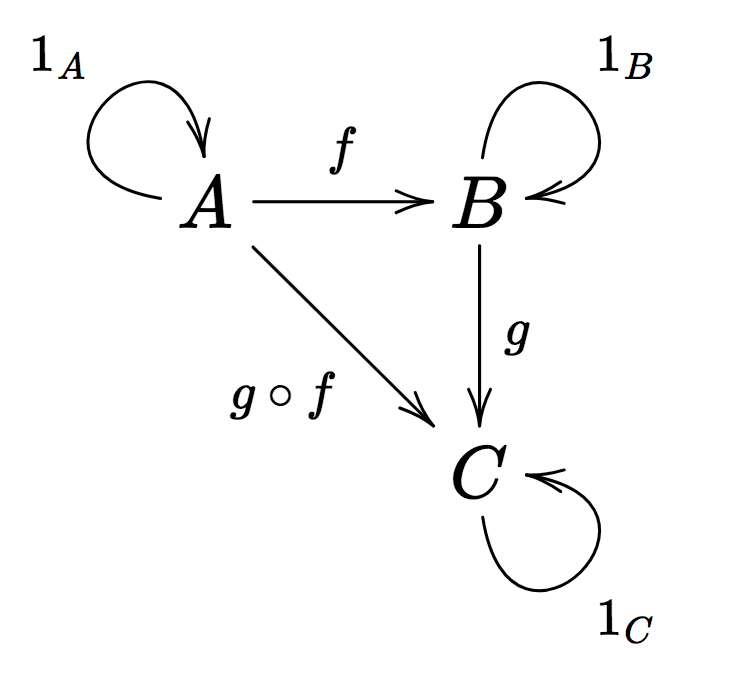Examples:

• Objects preserved by morphisms:

• Sets
• Vector spaces, Topological spaces, Groups, Rings, Manifolds…
• Logical formulas, Types (λ-calculus), …
• Structures forming one category:

• Posets, Monoids, Groups, Groupoids, …

### even (small) categories themselves form a category!

• Objects: Categories
• Morphisms: Functors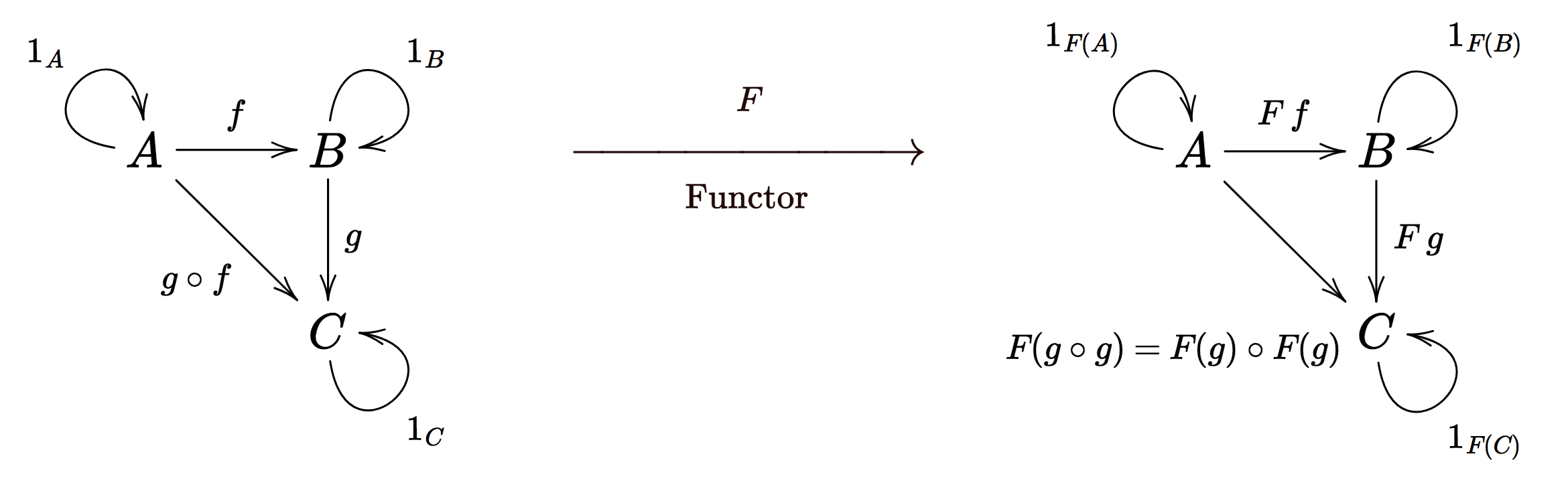### … as well as

Event structures and rigid maps:     $\ES$
Its (full) subcategory of finite posets:     $\Path$

Notation: $I : \Path \hookrightarrow \ES$ is its full embedding in $\ES$.

## Domains

Scott domains: $x ≤ y$ ⟺ the information carried by $y$ "extends" the one of $x$

### Category of finitary prime algebraic domains: $\PAD$

Let $D$ be a poset.

$X \subseteq \carrier D$ is finitely bounded:
iff $∀ \, y \in \Pfin(\carrier X)$, $y$ has an upper bound in $D$.

$D$ is consistently complete:
iff every finitely bounded subset $X$ has a least upper bound (lub) $\lub X$ in $D$.

If $D$ is consistently complete,   $p \in \carrier D$ is a complete prime:
iff for all $X \subset \carrier D$ bounded:
$$p \leq \lub X \implies \exists d \in X; \; p \leq d$$

Notation: $\dcp d ≝ \underbrace{\set{p \leq d \; \suchthat \; p \text{ complete prime}}}_{\text{prime downward-closure}}$

A prime algebraic domain:
is a consistently complete poset $D$ that satisfies the prime algebraicity property: for every $d \in \carrier D, \; d = \lub \dcp d$.

### Example: Posets and their complete primes (circled)Other examples and non-examples:

• $\unit ≝ [0,1]$ is not a prime algebraic domain.
• the ordinal $\omega + 1 := \set{0 \leq 1 \leq 2 \leq \cdots \leq \omega}$ is a prime algebraic domain.
• ${(\omega + 1)}^2$ (component-wise order) is a prime algebraic domain

Finitary prime algebraic domain:
if $\dcp p$ is finite for every complete prime $p$.

### Prime algebraic domain morphisms

Map of prime algebraic domains $f : C \to D$:
is a monotone function such that for every finitely bounded $X \subset \carrier C$, $\lub f[X] = f\,\lub X$.Generalised Scott continuous functions: preserve the lubs of directed sets, but also those of finitely bounded sets.

Notation: Category of finitary prime algebraic domains and their maps: $\PAD$

## Event structures as Finitary prime algebraic domains

Lemma (from event structures to prime algebraic domains): Let $E$ be an event structure. The poset $\pair{\ConfStar[E]}{\subset}$ is a prime algebraic domain, whose complete primes are the configurations $\lbrace \dc e \rbrace_{e ∈ \carrier E}$.

Conversely:

Lemma (from prime algebraic domains to event structures): Let $D$ be a finitary prime algebraic domain, we have an event structure $\CP_D$ given by:

• $\carrier {\CP_D} := \set{p \in \carrier D \; \suchthat \; p \text{ complete prime}}$
• $p_1 \leq_{\CP_D} p_2 \iff p_1 \leq_D p_2$
• $x \in \Con[\CP_D] \iff x \text{ bounded in } \carrier D$

Lastly, going back and forth between these two constructions:

Lemma:

$$∀ \, E ∈ \ES, \; E ≅ \CP_{\ConfStar[E]} \quad \qquad \quad ∀ \, D ∈ \PAD, \; D ≅ \pair{\ConfStar[\CP_D]}{\subset}$$

### Playing child: Finitary prime algebraic domain of configurations (complete primes underlined)# Event Structures as Presheaves

Presheaf over a category $\catC$:
a functor from a category $\opposite\catC$ to $\Set$
Natural transformations:
Functor morphisms

Yoneda embedding:

$$\yoneda_{\catC}: \begin{cases} \catC \to \overbrace{\Psh{\catC} ≝ [\opposite\catC, \Set]}^{\smash{\text{Category of Presheaves}}} \\ C \mapsto \Hom[\catC]{-, C} \end{cases}$$

Yoneda Lemma: For every presheaf $P ∈ \Psh{\catC}$, there is an isomorphism:

\begin{align*} &\Hom[\Psh{\catC}]{\yoneda_\catC(C), P} ≅ P(C) && \text{(natural in } C, P\text{)}\end{align*}

Examples: Cayley’s theorem, Dedekind–MacNeille completion, Skip-gram Model in ML, …

## Universal constructions

Natural transformations ≃ functor morphisms

#### Examples

Limits:
• Terminal objects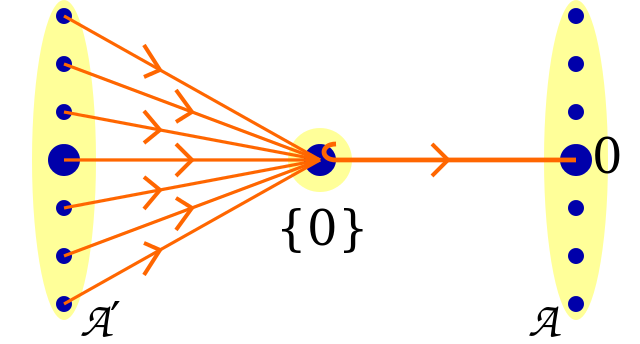• Products• Equalizers (Kernels), Pullbacks, …

Colimits:
• Initial objects

• Coproducts• Coequalizers (Quotients), Pushouts, …

A colimit of a functor $D: I ⟶ \C$ (called a diagram) is an initial object in the category of natural transformations from $D$ to a constant functor (the category of cocones).

Category of elements $\elem P$ of a presheaf $P ∈ \Psh\catC$:
• objects are pairs $\pair C x$, where $C ∈ \catC$ and $x ∈ PC$
• morphisms $\pair C x → \pair C {x'}$ are $\catC$-morphisms $f: C → C'$ such that $P f (x') = x$

## Presheaves as cocompletion

Let $\C$ be a small category and $\yoneda_\C: \C ⟶ \Psh\C$ its Yoneda embedding.

Theorem (Free Cocompletion of $\C$):

For every cocomplete category $\catD$ and functor $F :\C \to \catD$, there is a unique (up to isomorphism) cocontinuous functor $\hat F: \Psh\C \to \catD$ making the following triangle commute up to natural isomorphism:A puzzling question:

What does freely adding all its colimits to $\C$ have to do with presheaves over $\C$?

It can be shown that every diagram $D$ is "equivalent" to a diagram $D'$ that has the property:

$$\Comma{\yoneda_\C {D'}}{P_{D'}} ≅ \overbrace{\Comma{\yoneda_\C}{P_{D'}}}^{≅ \, \elem {P_{D'}}}$$

And the freely added colimit of $D$ in $\Psh\C$ will be taken to be the presheaf $P_{D'}$.

Any diagram $D: I ⟶ \C$ is "equivalent" to the diagram $\elem(\colim \yoneda_\C D) \xto U \C$, where $U$ is the forgetful functor. Thus:

$${P_D ≅ \colim\Bigg(\underbrace{\elem(\underbrace{\colim \yoneda_\C D}_{P_D})}_{≅ \, \Comma{\yoneda_\C}{P}} \xto{U}{\C} \xto{\yoneda_\C}\Psh\C\Bigg)}$$

Theorem (Every presheaf is a canonical colimit of representables): For all $P ∈ \Psh\C$,

$$P \, ≅ \, \colim\Big(\Comma{\yoneda_\C}{P} \xto U \C \xto {\yoneda_\C} \Psh\C\Big)$$

### Density formula/co-Yoneda lemma

Different take on the matter: through the lens of coends, which are universal extranatural transformations from a constant functor.

Theorem (co-Yoneda lemma): For every presheaf $P ∈ \Psh\C$:

$$P ≅ \int^c Pc × \yoneda_\C c$$

This is a particular case of tensor product of functors:
if $F ∈ \Psh\C$ and $G: \C ⟶ \catD$ where $\catD$ is cocomplete:
$$F \otimes_{\C} G ≝ \int^c F(c) \cdot G(c)$$

### Tensor product intuition

Let’s picture

• $\C$-objects as nonshiny Lego blocks
• their corresponding representables as the shiny versions
• $\catD$-objects as real-world physical objects.

1. $F$ (colimit of representables) = a shiny Lego construction/gluing
2. $G$ turns each nonshiny Lego block into a real-world $\catD$-object

Then

$F \otimes_{\C} G$ = real-world gluing where each shiny Lego block in $F$ is replaced by the image of the nonshiny corresponding Lego block by $G$.

The co-Yoneda lemma: $P \, ≅ \, P \otimes_{\C} \yoneda_\C$

Of course! Replacing each shiny Lego block in $P$ by itself yields $P$ again!

### Kan Extensions

Kan extensions: very expressive universal constructions that extend functors along one another.

« The notion of Kan extensions subsumes all the other fundamental concepts of category theory. » (MacLane)

Left Kan extension of a functor $F: \catC → \catD$ along a functor $K: \catC → \wC$:

is a functor $\Lan K F: \wC → \catD$ and a natural transformation $η: F → \Lan K F \circ K$ which is an initial arrow from $F ∈ [\catC, \catD]$ to $- \circ K: [\wC, \catD] → [\catC, \catD]$.

In other words: for any $G: \wC → \catD$ and $γ: F → GK$, there exists a unique natural transformation $α: \Lan K F → G$ such that $α_K \circ η = γ$:Theorem (Existence of Kan extensions along a functor into a cocomplete category):

Let $\C$ be a small category, and $K: \C → \wC, \, F: \C → \catD$ be functors.
If $\catD$ is cocomplete, $\Lan K F$ exists and can be defined, for all $\tilde C ∈ \wC$, as:

$$\Lan K F (\tilde C) ≝ \colim\Big(\Comma K {\tilde C} \xto U \C \xto F \catD\Big)$$

On top of that, if $F$ is fully faithful, the natural transformation $η: F → \Lan K F \circ K$ is an isomorphism.

With the machinery of Kan extensions, we have as corollaries:

• the fact that presheaves are colimits of representables

• the free cocompletion theorem

• the Yoneda and co-Yoneda lemmas (with Kan extensions as coends)

## Nerve construction

« The nerve construction is inherent in the theory of categories » (Tom Leinster)

Let $F: \C ⟶ \catD$ be a functor from a small category to locally small cocomplete one.

• The left Kan extension of $F$ along $\yoneda_\C$ is referred to as Yoneda extension or the realisation functor of $F$
• It has a right adjoint $\Nerve F ≝ \underbrace{D ⟼ \Hom[\catD]{F(-), D}}_{\text{denoted by } \Hom[\catD]{F(=), -}}$ called the nerve of $F$:$$\Lan{\yoneda_{\C}}{F} ⊣ \Nerve F \cong \Lan F {\yoneda_{\C}}$$

#### Nerve-Realisation paradigm intuition: let’s bring back our Lego blocks

You can think of

• $\C$-objects as being Lego blocks – and thus $\Psh\C$-objects
as being Lego constructions due to $\Psh\C$ being the free cocompletion of $\C$

• $\D$-objects as being real-world physical objects.

Then:

• the functor $F$ turns each Lego block into a real-world object.
• the realisation functor of $F$ takes a Lego construction and replaces each of its Lego block by their real-world counterpart given by $F$
• the nerve functor of $F$ associates to every real-world object the "closest matching" Lego construction for this object, by giving a way to probe the object with every Lego block.

For example, our child may have a bust of Newton in his bedroom (the bust being an object of $\catD$ in our comparison), and may
suddenly feel like making a Lego copy of it, thereby acting like the nerve: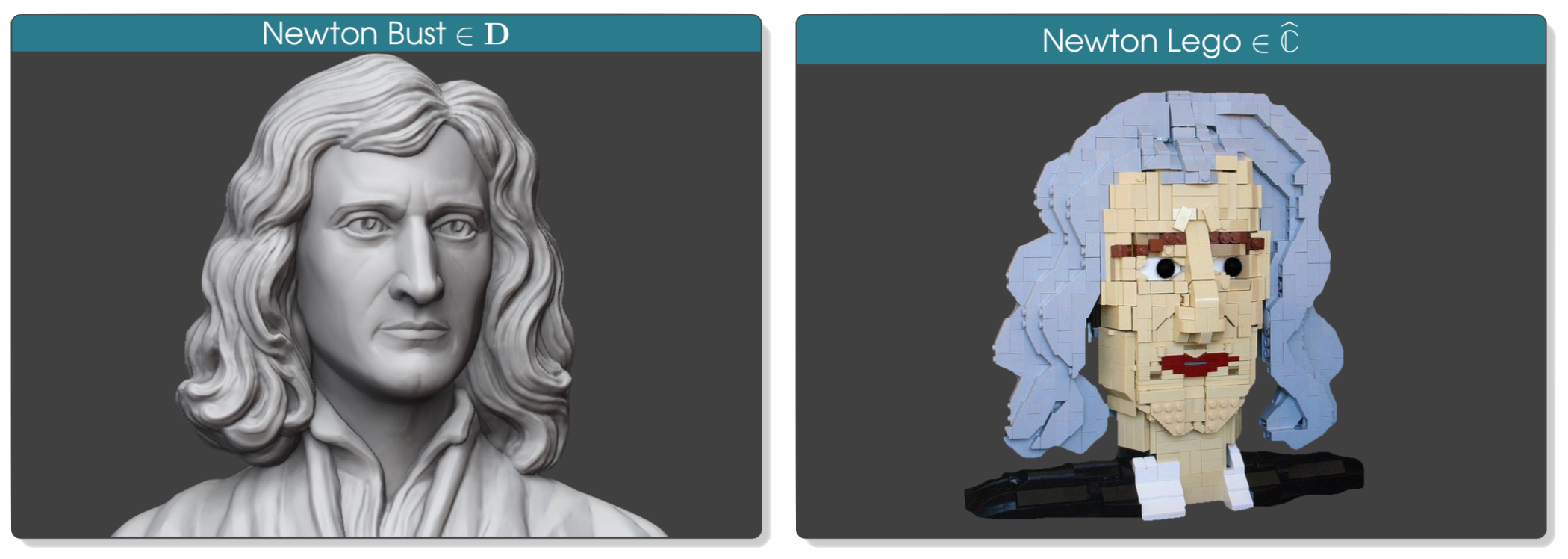## Nerve of the inclusion of finite paths into event structures

The nerve of the inclusion functor $\Path+ \stackrel{I_+}{\hookrightarrow} \ES$ enables us to
regard event structures as presheaves over non-empty paths.

$\Nerve {I_+}$ is fully faithful, due to $I_+$ being dense:

A functor $F: \catC ⟶ \catD$ is said to be dense/co-dense if for all $C ∈ \catC$:

$$C \; ≅ \; \colim\Big(\Comma F C \xto U \catC \xto F \catD\Big) \; / \; C \; ≅ \; \lim\Big(\Comma C F \xto U \catC \xto F \catD\Big)$$

and the fact that:

If $\C \stackrel{i}{\hookrightarrow} \catD$ is dense, the nerve functor $\Nerve i$ is fully faithful.

… that we can obtain as a corollary of this theorem:

Theorem: If $F: \catC → \catD$ is a functor, $G: \catC → \catD$ a continuous functor and $\catA \overset{i}{\hookrightarrow} \catC$ a co-dense subcategory, there is a unique extension of every natural transformation $αi: Fi → Gi$ to a natural transformation $α: F → G$.

To reuse the Lego analogy, $\C$ being dense in $\catD$ can be understood as the the Lego bricks being so small (let’s say of atomic size!) that the Lego constructions are faithful enough to distinguish any two non-isomorphic real-world objects!